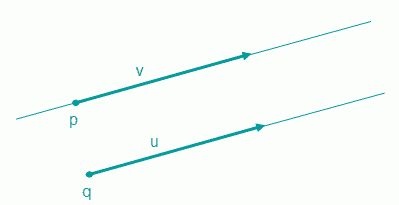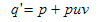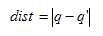Prev: Vector Projection Next: Plane

The line parametric equation is based on a point and a vector. The line will contain the point p, and the direction is provided by the vector v. The following figure illustrates this:The following equation can be used to provide points in the line:

`    point(t) = p + t * v`

where t is a scalar. Varying t we can obtain all points in the line. If t = 0, then the point returned is p. Other non-zero values for t will give us other points in the line.

The parametric definition can also be achieved using two points, p and p1, in which case a vector must be created from the two points, i.e. v = p1p

The difference between a line and a ray is that the former extends in both directions, where as the latter has a starting point and extends only in one direction.The figure above shows a line on the top and a ray on the bottom. Regarding the equation presented above, the difference between a line and a ray is the set of possible values for t. In a line, any value for t is admissible, where as for a ray t must be non-negative.

Distance between a point and a line

The shortest distance between a point and a line is the length of a line segment, from the point in question to a point in the line, that is perpendicular to the line’s direction.

Consider a line defined by a point p and a vector v. The goal is to compute the distance from point q to the line.The figure shows that the distance from point q to the line is the distance from q to q’. The point q’ can be determined by projecting the vector u on vector v:Hence, q’ can be determined as:So now the distance can be computed as:Distance between a point and a ray

As expected the formula is the same for points that project on the ray, but it is not always applicable as shown in the next figure.The formula presented for the distance between point and line only makes sense if the point projects in the ray, hence this situation should be tested when working with rays. A dot product between u and v will do, as the cosine of the angle will be negative when the point does not project on the ray.

If the point does not project on the ray, i.e. if the cosine is negative, then it may still make sense to know how far apart the point is from the ray. This is easily done computing the distance from p to q directly.

 Prev: Vector Projection Next: Plane

This site uses Akismet to reduce spam. Learn how your comment data is processed.